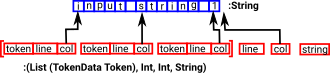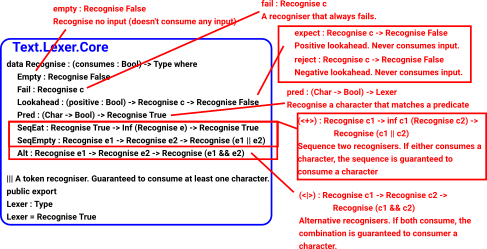# Lexer¶

The lexer is run by calling the ‘lex’ function in the Text.Lexer.Core module:

lex : TokenMap a -> String -> (List (TokenData a), (Int, Int, String))

 This function takes a String and returns a list of tokens. With the tokens we have indexes back to the original string which can be used in error messages:## TokenMap¶

In order to construct this list of tokens we need:

• A way to recognise the tokens in the input string.
• A way to construct these individual tokens.

This is given by the TokenMap which is a list of pairs containing this information:

(Lexer, String -> tokenType)


So for each Lexer in the list, if a substring in the input matches, run the associated function to produce a token of type tokenType

 from Text.Lexer.Core : https://github.com/idris-lang/Idris-dev/blob/master/libs/contrib/Text/Lexer/Core.idr TokenMap : (tokenType : Type) -> Type TokenMap tokenType = List (Lexer, String -> tokenType)  We can create a tokenMap by using a function like this: myTokenMap : TokenMap Token myTokenMap = [(is 'a',CharLit)] 

Once we have a TokenMap we can use it to lex many strings.

## Recognisers¶

The first part of each entry in the TokenMap is the recogniser.

A simple recogniser is ‘Pred’ which uses a predicate (Char -> Bool) to test whether to accept the character. It can be constructed using the ‘is’ function:

*parserEx> is 'a'
Pred (\ARG =>
intToBool (prim__eqChar ARG 'a'))
: Recognise True


More complicated structures can be recognised as tokens, this is done using the combinator structure of recognisers. This allows recognisers to be combined, for example,

 <+> means sequence two recognisers. If either consumes a character, the sequence is guaranteed to consume a character. *parserEx> is 'a' <+> is 'b' SeqEat (Pred (\ARG => intToBool (prim__eqChar ARG 'a'))) (Delay (is 'b')) : Recognise True  <|> means if both consume, the combination is guaranteed to consumer a character: *parserEx> is 'a' <|> is 'b' Alt (Pred (\ARG => intToBool (prim__eqChar ARG 'a'))) (Pred (\ARG => intToBool (prim__eqChar ARG 'b'))) : Recognise True 

This diagram shows the recogniser combinator with some of its constructors:A recogniser consumes its input, that is it advances the index to its input string, as it generates the token list. In some cases we don’t want this to happen, for instance, we may need to lookahead before consuming the input string.

If a recogniser does not consume input there is a danger of an infinite loop. To prevent this the recogniser is dependent on a boolean called ‘consumes’ which is true if the recogniser is guaranteed to consume at least one character.

The constructors of Recognise allow us to ensure that, even though parts of the recogniser may not consume, overall the recogniser will consume.

Since ‘Recognise True’ is more common than ‘Recognise False’ it is convenient to use ‘Lexer’ as a type synonym for ‘Recognise True’.

An important reason to have a lexer is to simplify the parser when whitespace or comments are required. Without this the parser would require a match for every combination, with and without, whitespace and comments.

In some languages the lexer needs to remove whitespace and comments so that they don’t appear in the token list. In some cases whitespace is significant but a single whitespace token can stand for any number of spaces, tabs, carriage returns and so on.

In some cases, such as when parsing the Idris language itself, whitespace is important to indicate blocks. In this case we might want to output a start-of-block token when the indent increases and an end-of-block token when the indent decreases.

## Simple Expression Example for Lexer¶

On this page we will implement a lexer to ‘lex’ a very simple expression as a running example, on the next page, we will go on to implement a parser for it.

First import the lexer and parser code:

module ParserExample

import Text.Lexer
import public Text.Parser.Core
import public Text.Parser


Then decide on the tokens that are needed. For this example the idea is to be able to parse simple arithmetic expressions, like this:

1+(2*3)


so we need:

• Numbers (for now integer literals are good enough).
• Some operators (for now +, - and * will do.
• Opening and closing Parentheses.

We can define these, as tokens, like this:

%default total

public export
data ExpressionToken = Number Integer
| Operator String
| OParen
| CParen
| EndInput


It may help with debugging and to implement error messages to implement show for these tokens:

export
Show ExpressionToken where
show (Number x) = "number " ++ show x
show (Operator x) = "operator " ++ x
show OParen = "("
show CParen = ")"
show EndInput = "end of input"

export
Show (TokenData ExpressionToken) where
show (MkToken l c t) = "line=" ++ show l ++ " col=" ++ show c ++ "tok=" ++ show t


The following defines the ‘TokenMap’ for this example:

||| integer arithmetic operators plus, minus and multiply.
export
opChars : String
opChars = "+-*"

operator : Lexer
operator = some (oneOf opChars)

toInt' : String -> Integer
toInt' = cast

expressionTokens : TokenMap ExpressionToken
expressionTokens =
[(digits, \x => Number (toInt' x)),
(operator, \x => Operator x),
(is '(' ,\x => OParen),
(is ')' ,\x => CParen)]


This specifies the lexer. It gives, for each token, a function to recognise the token type and a function to construct the token.

The library module ‘Text.Lexer’ contains useful functions to help with this. For example, the digits function used above, which reads one or more numeric characters.

We can now run the code with various strings to see what output the lexer produces. This is done by calling the ‘lex’ function with the TokenMap and input string as parameters:

cd Idris-dev/libs/contrib
idris -p contrib parserEx.idr
____    __     _
/  _/___/ /____(_)____
/ // __  / ___/ / ___/     Version 1.3.3
_/ // /_/ / /  / (__  )      http://www.idris-lang.org/
/___/\__,_/_/  /_/____/       Type :? for help

Idris is free software with ABSOLUTELY NO WARRANTY.
For details type :warranty.
Type checking ./Text/Token.idr
Type checking ./Text/Quantity.idr
Type checking ./Control/Delayed.idr
Type checking ./Data/Bool/Extra.idr
Type checking ./Text/Lexer/Core.idr
Type checking ./Text/Lexer.idr
Type checking ./parserEx.idr

*parserEx> lex expressionTokens "1+2"
([MkToken 0 0 (Number 1),
MkToken 0
(case fspan (\ARG => not (intToBool (prim__eqChar ARG '\n'))) "1" of
(incol, "") => c + cast (length incol)
(incol, b) => cast (length incol))
(Operator "+"),
MkToken 0
(case fspan (\ARG => not (intToBool (prim__eqChar ARG '\n'))) "+" of
(incol, "") => c + cast (length incol)
(incol, b) => cast (length incol))
(Number 2)],
0,
case fspan (\ARG => not (intToBool (prim__eqChar ARG '\n'))) "2" of
(incol, "") => c + cast (length incol)
(incol, b) => cast (length incol),
getString (MkStrLen "" 0)) : (List (TokenData ExpressionToken),
Int,
Int,
String)


The lexer uses potentially infinite data structures. It has recursive arguments (codata type) so code is lazy. In the output above the indexes have not been computed but we can pick out the tokens:

• (Number 1)
• (Operator “+”)
• (Number 2)

So the code is working.

We can now go ahead and parse this token list. A parser for this example will be constructed on the next page.